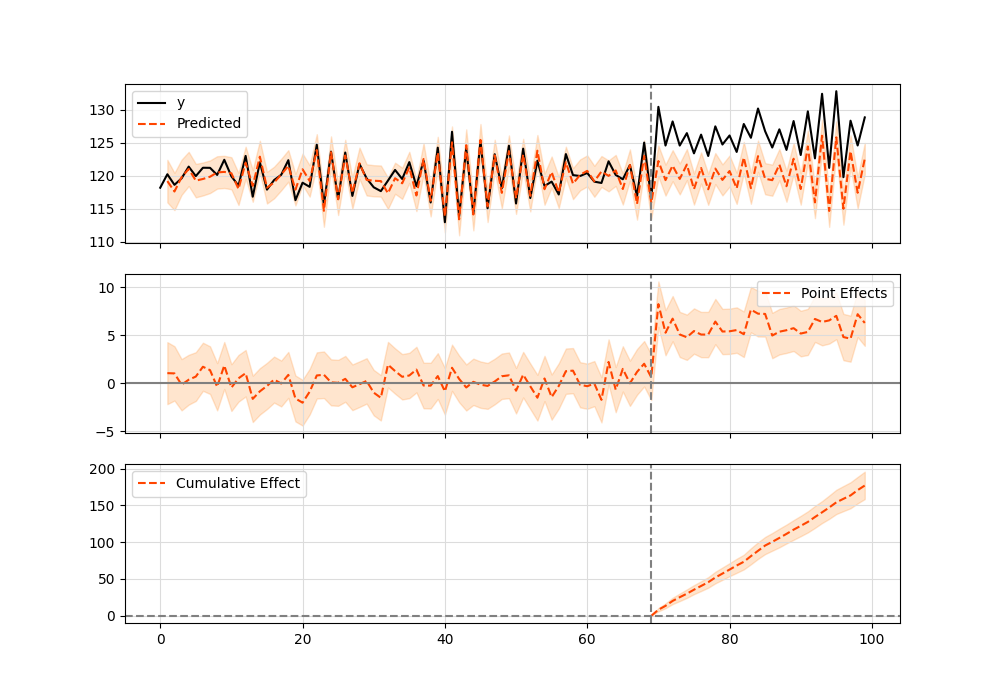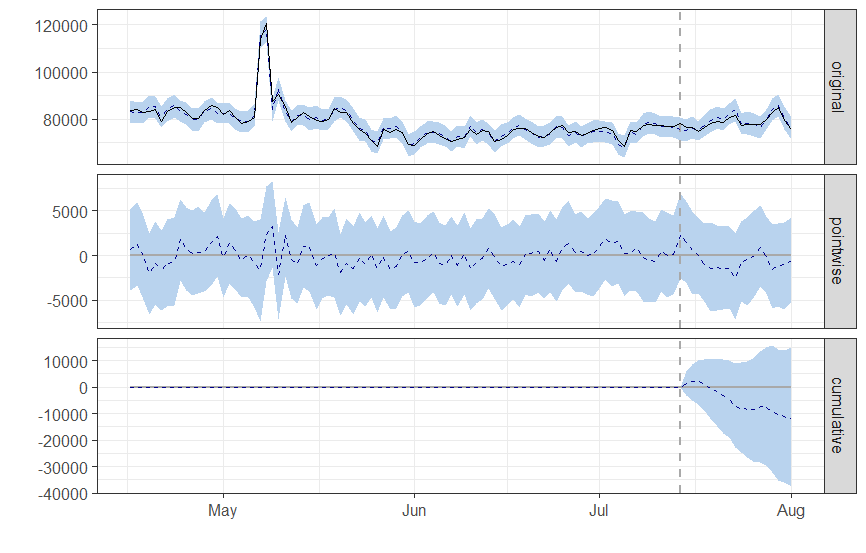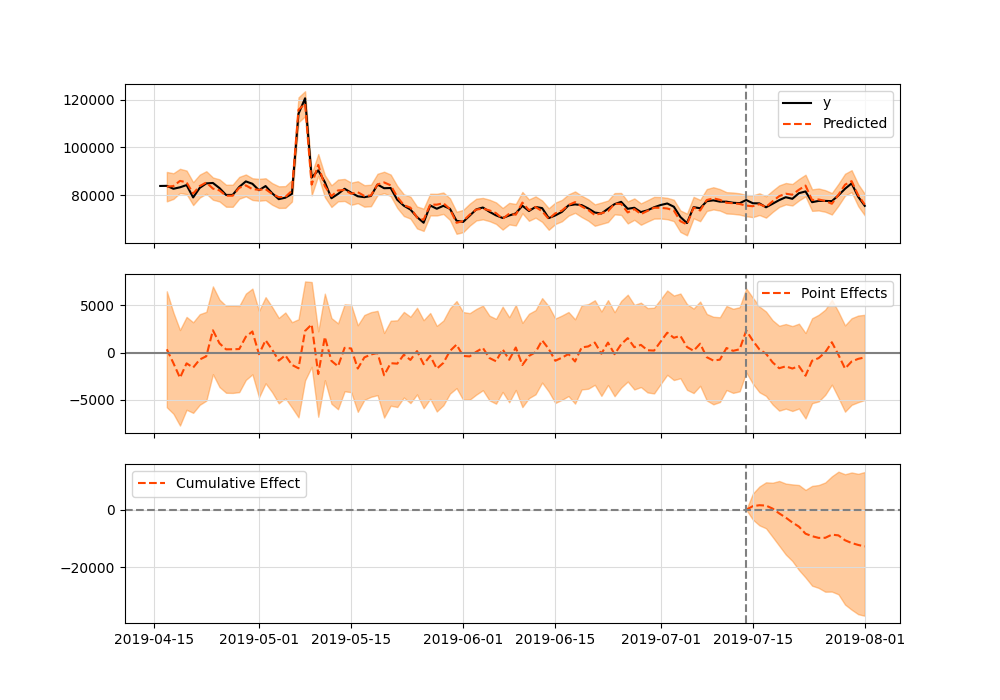# Tfcausalimpact

Python Causal Impact Implementation Based on Google's R Package. Built using TensorFlow Probability.
Alternatives To Tfcausalimpact
Probability3,882126213a day ago43June 07, 2022623apache-2.0Jupyter Notebook
Probabilistic reasoning and statistical analysis in TensorFlow
Ml Foundations1,705
5 months agomitJupyter Notebook
Machine Learning Foundations: Linear Algebra, Calculus, Statistics & Computer Science
Pymc4708
a year ago2January 25, 202049apache-2.0Jupyter Notebook
Experimental PyMC interface for TensorFlow Probability. Official work on this project has been discontinued.
Wtte Rnn58013 years ago5June 21, 201833mitPython
WTTE-RNN a framework for churn and time to event prediction
Tfcausalimpact36633 months ago20May 03, 202225apache-2.0Python
Python Causal Impact Implementation Based on Google's R Package. Built using TensorFlow Probability.
3 years ago3mitPython
TensorFlow implementation of GoogLeNet and Inception for image classification.
Deeplearningwithtf2.0227
3 years agoJupyter Notebook
Practical Exercises in TensorFlow 2.0 for Ian Goodfellows Deep Learning Book
Rethinking Tensorflow Probability207
a year agoapache-2.0Jupyter Notebook
Statistical Rethinking (2nd Ed) with Tensorflow Probability
Fenchel Young Losses175
5 months agoPython
Probabilistic classification in PyTorch/TensorFlow/scikit-learn with Fenchel-Young losses
C3d Keras159
6 years ago5otherPython
C3D for Keras + TensorFlow
Alternatives To Tfcausalimpact
Select To Compare

Alternative Project Comparisons

# tfcausalimpact

Google's Causal Impact Algorithm Implemented on Top of TensorFlow Probability.

## How It Works

The algorithm basically fits a Bayesian structural model on past observed data to make predictions on what future data would look like. Past data comprises everything that happened before an intervention (which usually is the changing of a variable as being present or not, such as a marketing campaign that starts to run at a given point). It then compares the counter-factual (predicted) series against what was really observed in order to extract statistical conclusions.

Running the model is quite straightforward, it requires the observed data `y`, covariates `X` that helps the model through a linear regression, a `pre-period` interval that selects everything that happened before the intervention and a `post-period` with data after the "impact" happened.

Please refer to this medium post for more on this subject.

## Installation

``````pip install tfcausalimpact
``````

## Requirements

• python{3.7, 3.8, 3.9, 3.10}
• matplotlib
• jinja2
• tensorflow>=2.10.0
• tensorflow_probability>=0.18.0
• pandas >= 1.3.5

## Getting Started

We recommend this presentation by Kay Brodersen (one of the creators of the Causal Impact in R).

We also created this introductory ipython notebook with examples of how to use this package.

This medium article also offers some ideas and concepts behind the library.

### Example

Here's a simple example (which can also be found in the original Google's R implementation) running in Python:

``````import pandas as pd
from causalimpact import CausalImpact

data.iloc[70:, 0] += 5

pre_period = [0, 69]
post_period = [70, 99]

ci = CausalImpact(data, pre_period, post_period)
print(ci.summary())
print(ci.summary(output='report'))
ci.plot()
``````

Summary should look like this:

``````Posterior Inference {Causal Impact}
Average            Cumulative
Actual                    125.23             3756.86
Prediction (s.d.)         120.34 (0.31)      3610.28 (9.28)
95% CI                    [119.76, 120.97]   [3592.67, 3629.06]

Absolute effect (s.d.)    4.89 (0.31)        146.58 (9.28)
95% CI                    [4.26, 5.47]       [127.8, 164.19]

Relative effect (s.d.)    4.06% (0.26%)      4.06% (0.26%)
95% CI                    [3.54%, 4.55%]     [3.54%, 4.55%]

Posterior tail-area probability p: 0.0
Posterior prob. of a causal effect: 100.0%

For more details run the command: print(impact.summary('report'))
``````

And here's the plot graphic:## Google R Package vs TensorFlow Python

Both packages should give equivalent results. Here's an example using the `comparison_data.csv` dataset available in the `fixtures` folder. When running CausalImpact in the original R package, this is the result:

### R

``````data = read.csv.zoo('comparison_data.csv', header=TRUE)
pre.period <- c(as.Date("2019-04-16"), as.Date("2019-07-14"))
post.period <- c(as.Date("2019-07-15"), as.Date("2019-08-01"))
ci = CausalImpact(data, pre.period, post.period)
``````

Summary results:

``````Posterior inference {CausalImpact}

Average          Cumulative
Actual                   78574            1414340
Prediction (s.d.)        79232 (736)      1426171 (13253)
95% CI                   [77743, 80651]   [1399368, 1451711]

Absolute effect (s.d.)   -657 (736)       -11831 (13253)
95% CI                   [-2076, 832]     [-37371, 14971]

Relative effect (s.d.)   -0.83% (0.93%)   -0.83% (0.93%)
95% CI                   [-2.6%, 1%]      [-2.6%, 1%]

Posterior tail-area probability p:   0.20061
Posterior prob. of a causal effect:  80%

For more details, type: summary(impact, "report")
``````

And correspondent plot:### Python

``````import pandas as pd
from causalimpact import CausalImpact

pre_period = ['2019-04-16', '2019-07-14']
post_period = ['2019-7-15', '2019-08-01']
ci = CausalImpact(data, pre_period, post_period, model_args={'fit_method': 'hmc'})
``````

Summary is:

``````Posterior Inference {Causal Impact}
Average            Cumulative
Actual                    78574.42           1414339.5
Prediction (s.d.)         79282.92 (727.48)  1427092.62 (13094.72)
95% CI                    [77849.5, 80701.18][1401290.94, 1452621.31]

Absolute effect (s.d.)    -708.51 (727.48)   -12753.12 (13094.72)
95% CI                    [-2126.77, 724.92] [-38281.81, 13048.56]

Relative effect (s.d.)    -0.89% (0.92%)     -0.89% (0.92%)
95% CI                    [-2.68%, 0.91%]    [-2.68%, 0.91%]

Posterior tail-area probability p: 0.16
Posterior prob. of a causal effect: 84.12%

For more details run the command: print(impact.summary('report'))
``````

And plot:Both results are equivalent.

## Performance

This package uses as default the `Variational Inference` method from `TensorFlow Probability` which is faster and should work for the most part. Convergence can take somewhere between 2~3 minutes on more complex time series. You could also try running the package on top of GPUs to see if results improve.

If, on the other hand, precision is the top requirement when running causal impact analyzes, it's possible to switch algorithms by manipulating the input arguments like so:

``````ci = CausalImpact(data, pre_period, post_period, model_args={'fit_method': 'hmc'})
``````

This will make usage of the algorithm `Hamiltonian Monte Carlo` which is State-of-the-Art for finding the Bayesian posterior of distributions. Still, keep in mind that on complex time series with thousands of data points and complex modeling involving various seasonal components this optimization can take 1 hour or even more to complete (on a GPU). Performance is sacrificed in exchange for better precision.

## Bugs & Issues

If you find bugs or have any issues while running this library please consider opening an `Issue` with a complete description and reproductible environment so we can better help you solving the problem.

Popular Probability Projects
Popular Tensorflow Projects
Popular Mathematics Categories
Related Searches

Get A Weekly Email With Trending Projects For These Categories
No Spam. Unsubscribe easily at any time.
Python
Algorithms